Courses

# Influence Lines for Beams (Part - 2) Civil Engineering (CE) Notes | EduRev

## Civil Engineering (CE) : Influence Lines for Beams (Part - 2) Civil Engineering (CE) Notes | EduRev

The document Influence Lines for Beams (Part - 2) Civil Engineering (CE) Notes | EduRev is a part of the Civil Engineering (CE) Course Structural Analysis.
All you need of Civil Engineering (CE) at this link: Civil Engineering (CE)

Instructional Objectives :

The objectives of the present lesson are as follows.

• Construction of influence line for maximum shear at sections in a beam supporting two concentrated loads
• Construction of influence line for maximum moment at sections in a beam supporting two concentrated loads
• Construction of influence line for maximum end shear in a beam supporting a series of moving concentrated loads
• Construction of influence line for maximum shear at a section in a beam supporting a series of moving concentrated loads
• Construction of influence line for maximum moment at a section in a beam supporting a series of moving concentrated loads
• Construction of influence line for absolute maximum moment in s beam supporting a series of moving concentrated loads
• Understanding about the envelopes of maximum influence line values

Introduction

In the previous lessons, we have studied about construction of influence line for the either single concentrated load or uniformly distributed loads. In the present lesson, we will study in depth about the beams, which are loaded with a series of two or more then two concentrated loads.

Maximum shear at sections in a beam supporting two concentrated loads

Let us assume that instead of one single point load, there are two point loads P1 and P2 spaced at y moving from left to right on the beam as shown in Figure 39.1. We are interested to find maximum shear force in the beam at given section C. In the present case, we assume that P2<P1.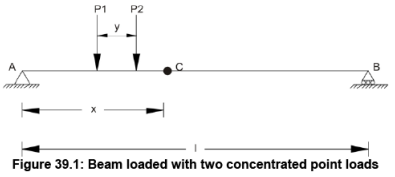Now there are three possibilities due to load spacing. They are: x<y, x = y and x>y.

Case 1: x<y

This case indicates that when load P2 will be between A and C then load P1 will not be on the beam. In that case, maximum negative shear at section C can be given by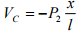and maximum positive shear at section C will be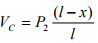Case 2: x=y

In this case, load P1 will be on support A and P2 will be on section C. Maximum negative shear can be given by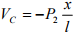and maximum positive shear at section C will be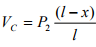Case 3: x>y

With reference to Figure 39.2, maximum negative shear force can be obtained when load P2 will be on section C. The maximum negative shear force is expressed as: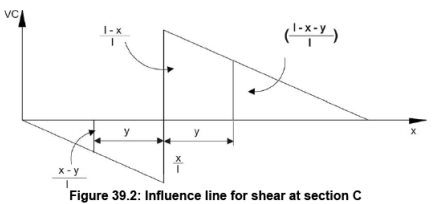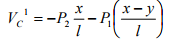And with reference to Figure 39.2, maximum positive shear force can be obtained when load P1 will be on section C. The maximum positive shear force is expressed as: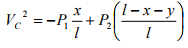From above discussed two values of shear force at section, select the maximum negative shear value.

Maximum moment at sections in a beam supporting two concentrated loads

Let us assume that instead of one single point load, there are two point loads P1 and P2 spaced at y moving left to right on the beam as shown in Figure 39.3. We are interested to find maximum moment in the beam at given section C.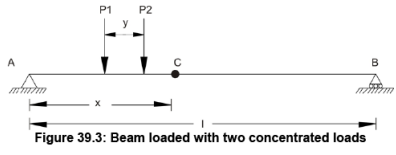With reference to Figure 39.4, moment can be obtained when load P2 will be on section C. The moment for this case is expressed as: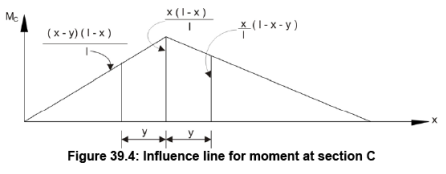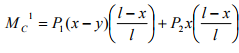With reference to Figure 39.4, moment can be obtained when load P1 will be on section C. The moment for this case is expressed as: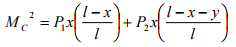From above two cases, maximum value of moment should be considered for maximum moment at section C when two point loads are moving from left end to right end of the beam.

Maximum end shear in a beam supporting a series of moving concentrated loads

In real life situation, usually there are more than two point loads, which will be moving on bridges. Hence, in this case, our aim is to learn, how to find end shear in beam supporting a series of moving concentrated loads. Let us assume that as shown in Figure 39.5, four concentrated loads are moving from right end to left end on beam AB. The spacing of the concentrated load is given in Figure 39.5.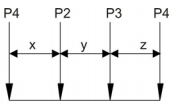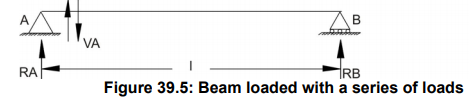As shown in figure, we are interested in end shear at A. We need to draw influence line for the support reaction A and a point away from the support at infinitesimal distance on the span for the shear VA. The influence lines for these cases are shown in Figure 39.6 and 39.7.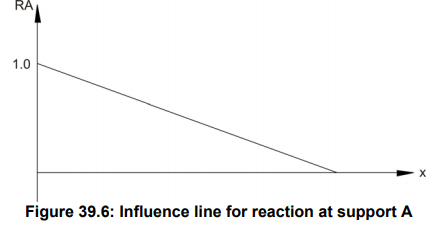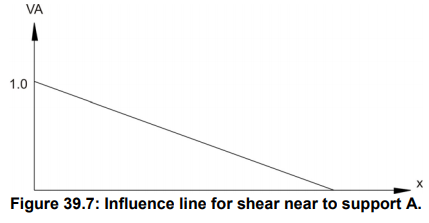When loads are moving from B to A then as they move closer to A, the shear value will increase. When load passes the support, there could be increase or decrease in shear value depending upon the next point load approaching support A. Using this simple logical approach, we will find out the change in shear value near support and monitor this change from positive value to negative value. Here for the present case let us assume that ΣP is summation of the loads remaining on the beam. When load P1 crosses support A, then P2 will approach A. In that case, change in shear will be expressed as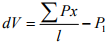When load P2 crosses support A, then P3 will approach A. In that case change in shear will be expressed as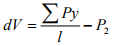In case if dV is positive then shear at A has increased and if dV is negative, then shear at A has decreased. Therefore, first load, which crosses and induces negative changes in shear, should be placed on support A.

Offer running on EduRev: Apply code STAYHOME200 to get INR 200 off on our premium plan EduRev Infinity!

## Structural Analysis

28 videos|114 docs|27 tests

,

,

,

,

,

,

,

,

,

,

,

,

,

,

,

,

,

,

,

,

,

;